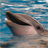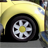QlikView App Dev

Discussion Board for collaboration related to QlikView App Development.

Announcements
QlikWorld 2022, LIVE in Denver CO., May 16-19, 2022. REGISTER NOW TO RECEIVE EARLY BIRD PRICING
cancel
Showing results for
Did you mean:Contributor II

Numbers of Days in selection

How calculate the number of calendar days in the period selections, regardless of the selections on the tables?

Thank You,

1 Solution

Accepted SolutionsMVP

May be this:

Max({1<Month = p(Month), Year = p(Year), Date = P(Date), Quarter = P(Quarter), MonthYear = p(MonthYear)>}Date) - Min({1<Month = p(Month), Year = p(Year), Date = P(Date), Quarter = P(Quarter), MonthYear = p(MonthYear)>}Date)

11 RepliesMVP

Can you elaborate on your requirement with an example?Specialist

Maybe This for Calendar Days

Num(Max(Datefield)-Min(Datefield))Specialist

Interval (Timestamp#(TimeEnd,'DD/MM/YYYY hh:mm:ss') - Timestamp#(TimeEnd,'DD/MM/YYYY

hh:mm:ss'), 'hh:mm:ss')Champion III

I'm guessing you mean the total number of calendar days in the data model, ignoring all selections, and calculated in script since this is in the scripting section?

[Days]:
LOAD max([Date]) - min([Date]) as [Days In Data Model]
;
AUTOGENERATE fieldvaluecount('Date')
;

But I probably wouldn't bother with script. I'd use the below expression, possibly as a result stored in a variable if I was using it in several places.

max({1} Date) - min({1} Date)Contributor II
Author

Thank you all for the reply.

Perhaps my question was not clear, and rephrase again:

I want to calculate the number of calendar days (in UI) corresponding selections of time (ex. Jan-april and year=2016),regardless of other selections they make (eg. Product, channel)?MVP

May be this:

Max({1<Month = p(Month), Year = p(Year), Date = P(Date), Quarter = P(Quarter), MonthYear = p(MonthYear)>}Date) - Min({1<Month = p(Month), Year = p(Year), Date = P(Date), Quarter = P(Quarter), MonthYear = p(MonthYear)>}Date)MVP

or this:

Max({1<Month = \$::Month, Year = \$::Year, Date = \$::Date, Quarter = \$::Quarter, MonthYear = \$::MonthYear>}Date) - Min({1<Month = \$::Month, Year = \$::Year, Date = \$::Date, Quarter = \$::Quarter, MonthYear = \$::MonthYear>}Date)Champion III

Do we need the p() for anything other than [Date]? All the other fields should have a direct effect on what values of [Date] are possible, and so I think selections in them would indirectly count. I may be missing something but it seems to be working on a simple example I'm fiddling with. So might this do the trick?

max({1<[Date]=p([Date])>} [Date]) -

min({1<[Date]=p([Date])>} [Date])Champion III

Wait, since other selections affect what is possible, neither approach works. I think we have to explicitly tell it which fields to ignore, not which ones to use. So... dollar sign expansion with a funky concat(). Hmmm... something like this, assuming all these fields are on a calendar connected only by Date to the rest of the model?

max({<[\$(=concat({<[\$Table]-={'Calendar'},[\$Field]-={'Date'}>} distinct [\$Field],']=,['))]=}>} [Date]) - min({<[\$(=concat({<[\$Table]-={'Calendar'},[\$Field]-={'Date'}>} distinct [\$Field],']=,['))]=}>} [Date])

I need to find an app with a calendar and see...

Edit: Yeah, that seems to work.Community Browser